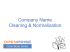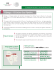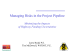# ILP Exercises Department of informatics Computer Science degree

## Transcription

ILP Exercises Department of informatics Computer Science degree
```Department of informatics
Computer Science degree
Computer Architecture
ILP Exercises
C.1 Use the following code fragment:
Loop: LD R1 ,0(R2)
S D Rl,0(R2)
DSUB R4,R3,R2
BNEZ R4, Loop
;R l = R l + l
;R2=R2+4
;R4zR3-R2
;branch to Loop if R4 ! = 0
Assume that the initial value of R3 is R2 + 396.
a.
b.
c.
d.
e.
f.
g.
Data hazards are caused by data dependences in the code. Whether a dependency causes a hazard depends on the machine
implementation (i.e., number of pipeline stages). List all of the data dependences in the code above. Record the register, source
instruction, and destination instruction;for example, there is a data dependency for register R1 from the LD to the DADDI.
Show the timing of this instruction sequence for the 5-stage RISC pipeline without any forwarding or bypassing hardware but
assuming that a register read and a write in the same clock cycle "forwards" through the register file, as shown in Figure C.6.
Use a pipeline timing chart like that in Figure C.5. Assume that the branch is handled by flushing the pipeline. If all memory
references take 1 cycle, how many cycles does this loop take to execute?
Show the timing of this instruction sequence for the 5-stage RISC pipeline with full forwarding and bypassing hardware. Use a
pipeline timing chart like that shown in Figure C.5. Assume that the branch is handled by predicting it as not taken. If all
memory references take 1 cycle, how many cycles does this loop take to execute?
Show the timing of this instruction sequence for the 5-stage RISC pipeline with full forwarding and bypassing hardware. Use a
pipeline timing chart like that shown in Figure C.5. Assume that the branch is handled by predicting it as taken. If all memory
references take 1 cycle, how many cycles does this loop take to execute?
High-performance processors have very deep pipelines-more than 15 stages. Imagine that you have a 10-stage pipeline in which
every stage of the 5-stage pipeline has been split in two. The only catch is that, for data forwarding, data are forwarded from the
end of a pair of stages to the beginning of the two stages where they are needed. For example, data are forwarded from the
output of the second execute stage to the input of the first execute stage, still causing a I-cycle delay. Show the timing of this
instruction sequence for the 10-stage RISC pipeline with full forwarding and bypassing hardware. Use a pipeline timing chart
like that shown in Figure C.5. Assume that the branch is handled by predicting it as taken. If all memory references take 1
cycle, how many cycles does this loop take to execute?
Assume that in the 5-stage pipeline the longest stage requires 0.8 ns, and the pipeline register delay is 0.1 ns. What is the
clock cycle time of the 5-stage pipeline? If the 10-stage pipeline splits all stages in half, what is the cycle time of the LOstage machine?
Using your answers from parts (d) and (e), determine the cycles per instruction (CPI) for the loop on a 5-stage pipeline
and a 10-stage pipeline. Make sure you count only from when the first instruction reaches the write-back stage to the end.
DO not count the start-up of the first instruction. Using the clock cycle time calculated in part (0, calculate the average
instruction execute time for each machine.
C.2 Suppose the branch frequencies (as percentages of all instructions) are as follows:
Conditional branches 15%
Jumps and calls 1 %
Taken conditional branches 60% are taken
a. We are examining a four-deep pipeline where the branch is resolved at the end of the second cycle for unconditional
branches and at the end of the third cycle for conditional branches. Assuming that only the first pipe stage can always be
done independent of whether the branch goes and ignoring other pipeline stalls, how much faster would the machine be
without any branch hazards?
b. Now assume a high-performance processor in which we have a 15-deep pipeline where the branch is resolved at the end
of the fifth cycle for unconditional branches and at the end of the tenth cycle for conditional branches. Assuming that
only the first pipe stage can always be done independent of whether the branch goes and ignoring other pipeline stalls,
how much faster would the machine be without any branch hazards?
Department of informatics
Computer Science degree
Computer Architecture
C.3 We begin with a computer implemented in single-cycle implementation. When the stages are split by functionality, the stages
do not require exactly the same amount of time. The original machine had a clock cycle time of 7 ns. After the stages were split,
the measured times were IF, 1 ns; ID, 1.5 ns; EX, 1 ns; MEM, 2 ns; and WB, 1.5 ns. The pipeline register delay is 0.1 ns.
a. What is the clock cycle time of the 5-stage pipelined machine?
b. If there is a stall every 4 instructions, what is the CPI of the new machine?
c. What is the speedup of the pipelined machine over the single cycle machine?
a. If the pipelined machine had an infinite number of stages, what would its speedup be over the single-cycle machine?
```

### Introduction to Computer Architecture Assignment 2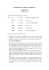### Empalme Gas Pipeline Branch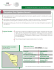### Hermosillo Gas Pipeline Branch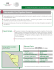### Samalayuca – Sasabe Gas Pipeline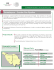### CMSC 411 : Homework #3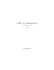### S. Ellis - Community Advisory Boards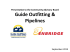### Wampee Conference Center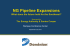### flyer - Centennial Classic Arms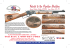### pETROSANTANdER (uSA) INC.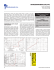### Company Name Cleansing And Normalization